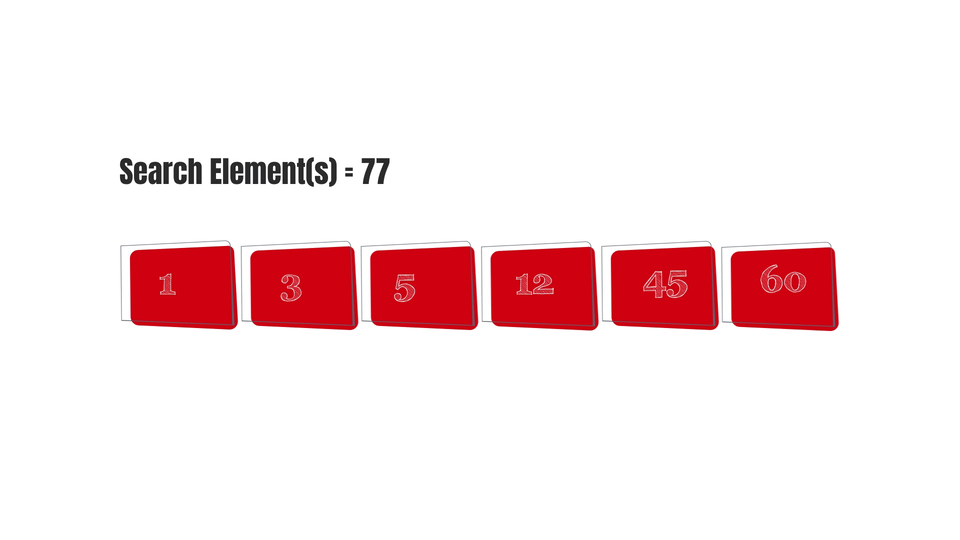# Binary SearchCreated August 8, 2021

Binary Searching algorithm is used to search for an element in a sorted array. It involves dividing an array into halves until the element is finally found.

I've already given a brief introduction of this algorithm in one of my previous posts. You can find it here if you haven't read that yet.

So let's get started with the algorithm now.

## The Algorithm

It's a little tricky to understand at first. Also, this algorithm is carried out recursively. So play a close attention to it -

1. Get an array(arr[]) and the search element(s) from the user.
2. Sort the array.
3. Set the Lower Limit (u) and Upper Limit(l). l-> the first index value. u-> the last index value.
4. Calculate the average of Upper and Lower limits. av = (u+l)/2
5. Set middle(or m) equal to av. It is the middle-most element of the array.
6. Now, check if the value of middle element(m) is greater than, equal to or less than the search element(s). If:
1. arr[m] > s - set upper limit as element just before the middle element.
2. arr[m] == s - then return the value of m as m is the element we were searching for.
3. arr[m] < s - set lower limit as element just after middle element(m).
7. Now repeat the steps from 4 to 6 again and again(recursion) while l<=u until l, u and m both point at a single element at the end.

## The Code

The C code for the above algorithm is -

``````int BinSearch(int *arr, int s, int l, int u){
if( l > u){//when lower limit becomes greater than upper limit
}

int m = (l + u)/2; //calculate average. The middle most index

if(s == arr[m]){//when mid element value is equal to search element
return m; //when element is found
}
else if(s < arr[m]){
BinSearch(arr, s, l, m-1); //when mid element value is greater than search element
}else{
BinSearch(arr, s, m+1, u); //when mid element value is less than search element
}
}
``````

The above code will only work if you enter the elements in ascending order of their values as Binary Search only works on sorted arrays. You can also make use of inbuilt sorting functions or apply sorting algorithms to sort the array.

The whole code is-

``````#include <stdio.h>
#include <stdlib.h>

int BinSearch(int *arr, int s, int l, int u){
if( l > u){//when lower limit becomes greater than upper limit
}

int m = (l + u)/2; //calculate average. The middle most index

if(s == arr[m]){//when mid element value is equal to search element
return m; //when element is found
}
else if(s < arr[m]){
BinSearch(arr, s, l, m-1); //when mid element value is greater than search element
}else{
BinSearch(arr, s, m+1, u); //when mid element value is less than search element
}
}

int main(){
int n, *arr, s;
printf("Enter number of elements: ");
scanf(" %d", &n);

arr = (int *)malloc(n*sizeof(int));
for(int i=0; i<n; i++){ //enter in increasing order
printf("Enter element: ");
scanf(" %d", &arr[i]);

printf("Enter the Search Element: ");
scanf(" %d", &s); // getting the search element

//set lower and upper limits
int l =0,  u = n-1;

//implement Binary Search
int ans = BinSearch(arr, s, 0, n-1);
if(ans == -1){
}else{
printf("Element found!\n");
}

}
``````

Here's a gif to make it clearer for you -